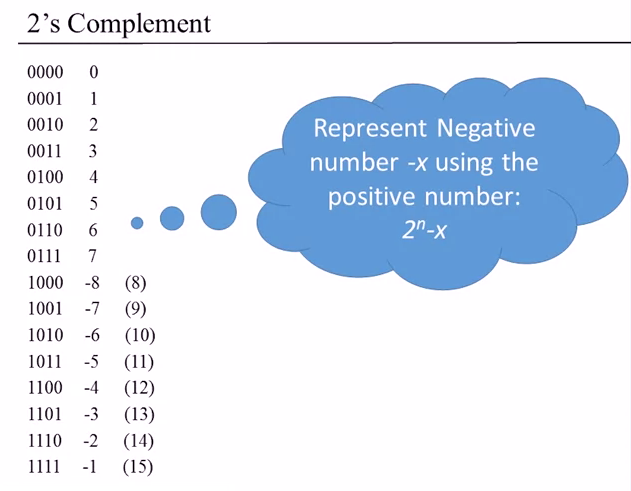# I have trouble understanding negative numbersClassicListThreaded3 messagesOpen this post in threaded view
|

## I have trouble understanding negative numbers

 This post was updated on . I have trouble understanding how this works:I don't understand the `- x` part. If I get that part right, `2^n` is 2^4 in this case, n being the word size (max number of bits we can use to represent the number). But then to represent -7, we'd have x = -7 so (2^n) - x => (2^4) - (-7) => 16 + 7 => 23 Or if we consider that x = 7 and -x = -7 (which is the number we want to represent) (2^4) - 7 => 16 - 7 => 9 So then we have to keep mapping positive numbers to their negative counterparts?
 Administrator wetFence wrote I have trouble understanding how this works: Or if we consider that x = 7 and -x = -7 (which is the number we want to represent) (2^4) - 7 => 16 - 7 => 9 So then we have to keep mapping positive numbers to their negative counterparts? The above is the correct interpretation. The confusion is that bit patterns with the most significant bit set have two different meanings. They can either mean large positive numbers or negative numbers. Some programming languages, like C, expose both of these natures of binary numbers. Others, like Java, only expose the signed values. The Hack computer, treats numbers as signed. The beauty of the 2's-complements system is that the Hack Computer's hardware doesn't need to do anything different to handle signed and unsigned numbers. For example: ```3 + -5 = -2 3 + 11 = 14 11 11 0011 0011 +1011 +1011 ---- ---- 1110 1110 ```The single-bit sums and carries are identical for both the signed and unsigned additions. When you design your Add16 you don't need to worry about whether the inputs are signed or unsigned. Do pay attention when you read about the ALU and note the difference between arithmetic negation and logical (bitwise) negation. --Mark _____  There are some subtleties to signed multiplication and overflow handling but the Hack computer doesn't have any of that hardware.